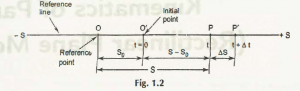Rectilinear motionis the term used for depicting a straight line, or to be specifically speaking, the path in which the particles are in motion.

In a straight line, ‘-S’ is used for depicting the initial point of that line whereas ‘S’ is used for showing the end of that line. A certain particle ‘r’ is seen moving along that straight line. In between the reference line with points ‘-S’ and ‘S,’there are 4 more points.Among those 4 points, O is taken as the initial position S = S0 and P at t = 0. In this case, P is the position of a particle at time ‘t.’

At a certain distance from the point P, there is another point P’.

For that position, its calculated time =t+ ∆t.

Displacement in ‘m’ for aunit time =∆S /∆t.

Displacement in time is ∆t =∆S.

In case of ∆t à 0, which is a limiting case, velocity is calculated as V = Lt

Average velocity is taken to be as ∆S /∆t = Vav

Equation for instantaneous velocity, which is also depicted as V is ∆S /∆t= dS /dt.

This again on equating comes to be as v = dS/dt =S.

The velocity when calculated at initial point O stands to be V = Vo. Time in this respect is 0.

After designating all the formulations, the definition of average acceleration which takes place during the time depicted by ∆t is aav =∆v / ∆t (equational term). In here, ‘a’ is defined as instantaneous acceleration of that particle.

In limiting case, the equation for it is a = dv /dt =v.

When considering for‘-a’, it is equivalent to retardation or instantaneous deceleration. So, on this context, a d2S / dt = S

From the first equation we have (dt = dS /v)

And from the second equation, we have (dt = dv / a)

Therefore, calculating with both equations, the final answers stands to be,

(dt = dS /v = dv /a)

And (v dv =a dS)

Links of Previous Main Topic:-

Links of Next Mechanical Engineering Topics:-

### Submit Your Assignment### Customer Reviews

My Homework Help
Rated 5.0 out of 5 based on 510 customer reviews at